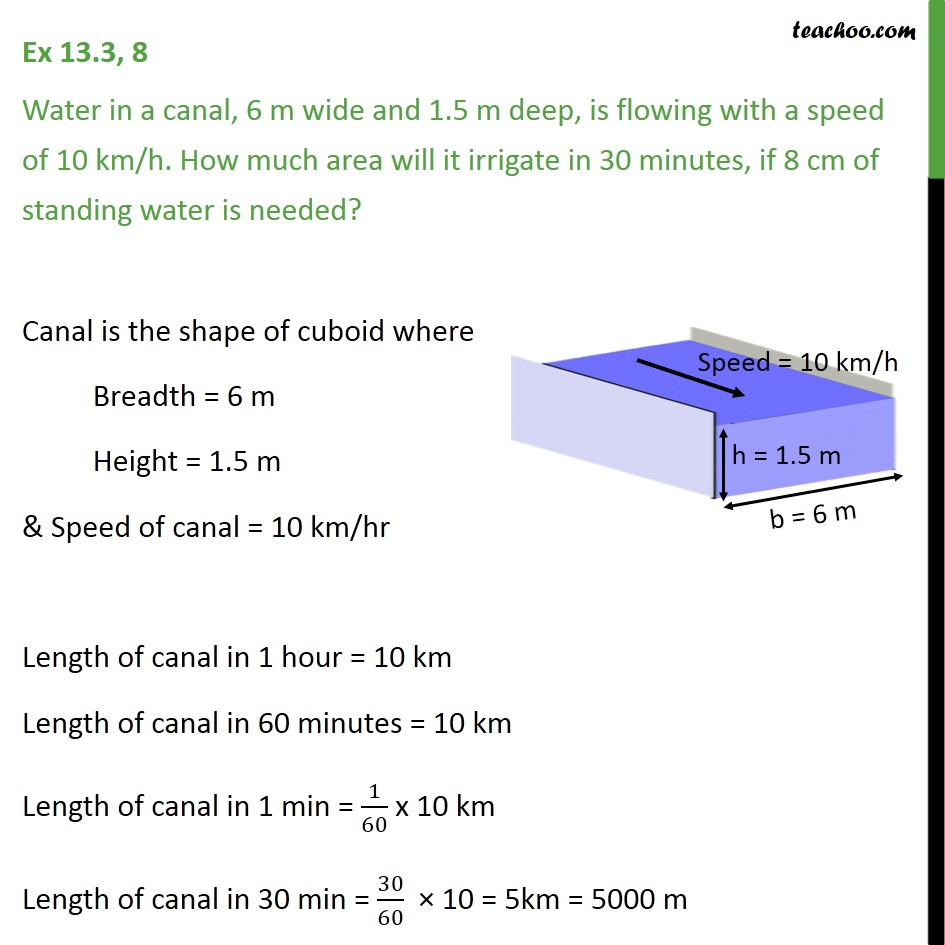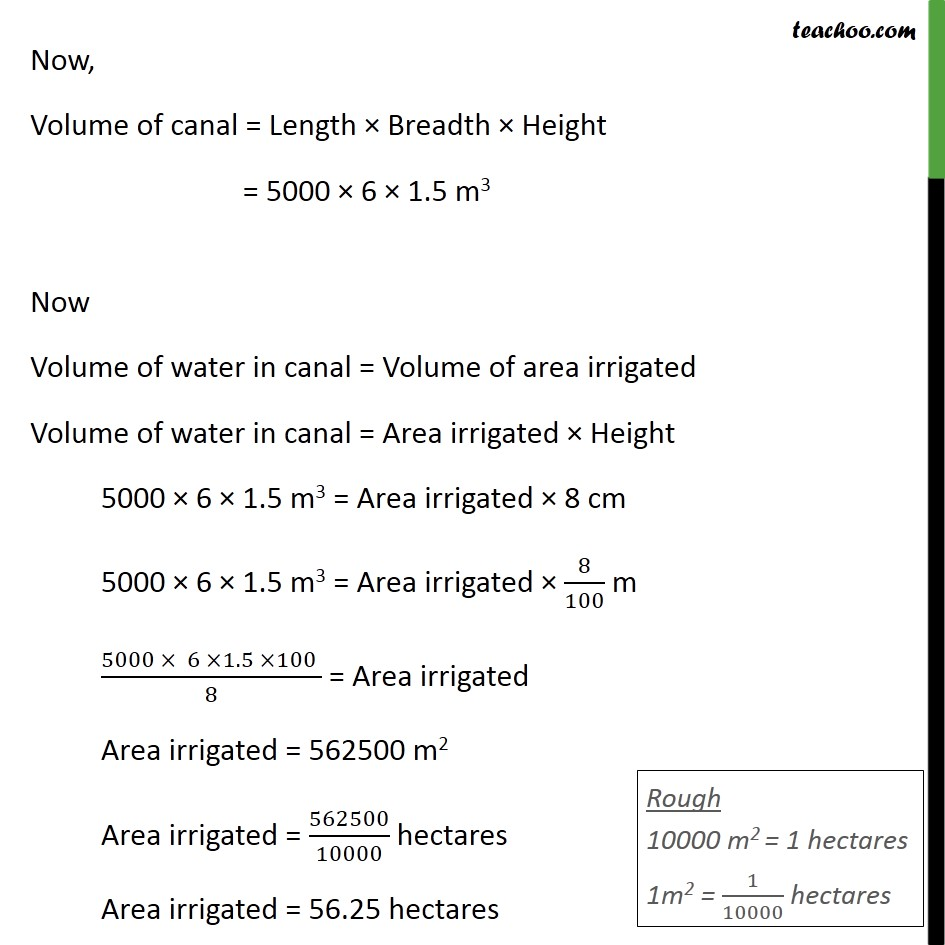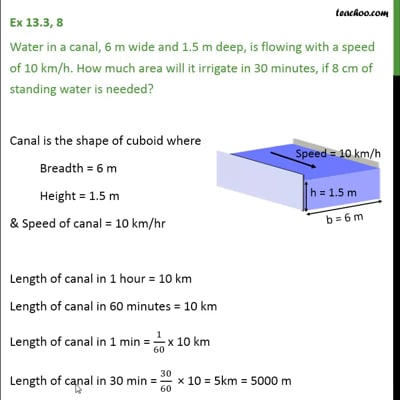Ex 13.3

Chapter 13 Class 10 Surface Areas and Volumes (Term 2)
Serial order wiseThis video is only available for Teachoo black users

### Transcript

Ex 13.3, 8 Water in a canal, 6 m wide and 1.5 m deep, is flowing with a speed of 10 km/h. How much area will it irrigate in 30 minutes, if 8 cm of standing water is needed? Canal is the shape of cuboid where Breadth = 6 m Height = 1.5 m & Speed of canal = 10 km/hr Length of canal in 1 hour = 10 km Length of canal in 60 minutes = 10 km Length of canal in 1 min = 1/60 x 10 km Length of canal in 30 min = 30/60 10 = 5km = 5000 m Now, Volume of canal = Length Breadth Height = 5000 6 1.5 m3 Now Volume of water in canal = Volume of area irrigated Volume of water in canal = Area irrigated Height 5000 6 1.5 m3 = Area irrigated 8 cm 5000 6 1.5 m3 = Area irrigated 8/100 m (5000 6 1.5 100 )/8 = Area irrigated Area irrigated = 562500 m2 Area irrigated = 562500/10000 hectares Area irrigated = 56.25 hectares